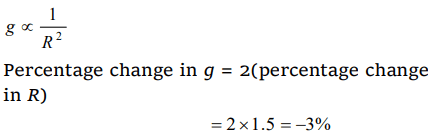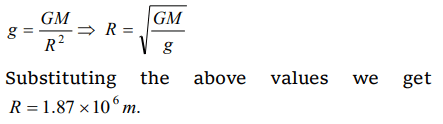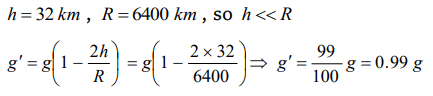## Gravitation Questions and Answers Part-7

1. Let g be the acceleration due to gravity at earth's surface and K be the rotational kinetic energy of the earth. Suppose the earth's radius decreases by 2% keeping all other quantities same, then
a) g decreases by 2% and K decreases by 4%
b) g decreases by 4% and K increases by 2%
c) g increases by 4% and K increases by 4%
d) g decreases by 4% and K increases by 4%

Explanation:2. Where will it be profitable to purchase 1 kilogram sugar
a) At poles
b) At equator
c) At 45° latitude
d) At 40° latitude

Explanation: Weight is least at the equator

3. If the radius of the earth shrinks by 1.5% (mass remaining same), then the value of acceleration due to gravity changes by
a) 1%
b) 2%
c) 3%
d) 4%

Explanation:4. If radius of the earth contracts 2% and its mass remains the same, then weight of the body at the earth surface
a) Will decrease
b) Will increase
c) Will remain the same
d) None of these

Explanation:5. If mass of a body is M on the earth surface, then the mass of the same body on the moon surface is
a) M/6
b) Zero
c) M
d) None of these

Explanation: Mass does not vary from place to place

6.Mass of moon is $7.34\times10^{22 }kg$   . If the acceleration due to gravity on the moon is $1.4m\diagup s^{2}$ , the radius of the moon is $\left(G=6.667\times10^{-11 }Nm^{2}\diagup kg^{2}\right)$
a) $0.56\times10^{4 }m$
b) $1.87\times10^{6 }m$
c) $1.92\times10^{6 }m$
d) $1.01\times10^{8 }m$

Explanation:7.What should be the velocity of earth due to rotation about its own axis so that the weight at equator become 3/5 of initial value. Radius of earth on equator is 6400 km
a) $7.4\times10^{-4 }rad\diagup sec$
b) $6.7\times10^{-4 }rad\diagup sec$
c) $7.8\times10^{-4 }rad\diagup sec$
d) $8.7\times10^{-4 }rad\diagup sec$

Explanation:8. Acceleration due to gravity is ‘g’ on the surface of the earth. The value of acceleration due to gravity at a height of 32 km above earth’s surface is (Radius of the earth = 6400 km)
a) 0.9 g
b) 0.99 g
c) 0.8 g
d) 1.01 g

Explanation:9. At what height from the ground will the value of ‘g’ be the same as that in 10 km deep mine below the surface of earth
a) 20 km
b) 10 km
c) 15 km
d) 5 km